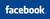EMI calculator for Home Loans

 Follow AllBankingSolutions  @# EMI Calculator - India

Best  EMI Calculator  for Home Loans, Auto Loans, Personal Loans by Banks in India like SBI, PNB, ICICI, Axis Bank etc.

EMI calculator below allows you to compare various options or quotes from different banks / financial institutions.

 Compare Your EMIs for House Loans, Vehicle Loans, Personal Loans with different Interest Rates and different  Repayment Periods Repayment Period (in Months) Rate of Interest Payable Loan Amount EMI or Monthly Repayment

The above EMI calculator gives a  close approximation of  loan repayments, but actual amount may differ from Bank to Bank, as different Banks sometimes use slightly different methods for EMI calculations.    Click below for a more detailed month wise repayment of Interest and Principal through monthly EMIs.

Q.1  :  What is an EMI ?

EMI means 'Equated Monthly Installments'.     Thus, EMI calculator gives you the amount that  you have to pay every month towards repayment of your loan.

Q.2 : What are the factors on which an EMI depends ?

EMI for a given loan amount depends on  (a) loan amount, (b) rate of interest and (c) tenure of the loan.     EMI will be lower if  one increases  the loan tenure / repayment period of the loan.

Q. 3: Do Banks Charge Interest towards beginning of Loan Period or Towards End of Loan Period ?

Each EMI  comprises of  (i) an interest component and  (ii) a principal component.    Thus, interest on loan is paid on a continuous basis.

Q. 4 : In which type of loans is EMI applicable ?

EMI is mainly used for retail loans  that are offered by all major banks in India.  (These banks use the same EMI formula for arriving at the monthly installments.   However, there can be minor variations owing to the method adopted for the initial payments or the timing of the installment during the month).

Q. 5 :  Why EMIs are Popular?

EMIs are popular both with the borrowers and lenders.  Borrowers prefer them as they know precisely how much money they will need to pay toward their loan each month.   This helps them making the personal monthly budgeting process easier.  Lenders are able to monitor the repayments easily and any default is repayment can be easily detected.

Q. 6 : How to Calculate my EMI for a Loan ?

EMI formula is a bit complex.   Even most of the bankers are not aware of the formula for calculation of the EMI.

Let us first understand how does the banks calculate interest and principal payments from each EMI.  The most important point you have to understand is that EMI consists of  repayment of  an unequal combination of principal and interest rate.    In the initial  years of loan repayment, interest payments for major portion of the EMI while the principal amount is much less. However, towards the end of the repayment tenure, it is more of the principal that is being repaid,  and only small portion is the interest.

Thus, though the EMI remains constant every month, one pays a higher component of interest when he / she began repaying the loan and a higher component of principal towards the end.

We will discuss below the formula and other excel utilities available for detailed calculation of EMIs.

 EMI Formula    We have already mentioned elsewhere that this formula is a bit complex.  A person with not much background of mathematics will find difficult to understand or make use of the same.  However, the mathematical  formula for calculation of EMI is as under :   EMI =    (p*r) (1+r)^n             ___________               (1+r)^n - 1 p = principal (amount of loan)  r = rate of interest per instalment period, i.e., if interest is 12% p.a. r = 1,  n = no. of installments in the tenure,  ^ denotes whole to the power.

EMI CALCULATOR IN EXCEL : CLICK HERE TO KNOW HOW TO CALCULATE EMI IN EXCEL